# Working with Geospatial Data in Python

• Difficulty Level : Expert
• Last Updated : 23 Aug, 2021

Spatial data, also known as geospatial data, GIS data, or geodata, is a type of numeric data that defines the geographic location of a physical object, such as a building, a street, a town, a city, a country, or other physical objects, using a geographic coordinate system. You may determine not just the position of an object, but also its length, size, area, and shape using spatial data.

To work with geospatial data in python we need the GeoPandas & GeoPlot library

Attention geek! Strengthen your foundations with the Python Programming Foundation Course and learn the basics.

To begin with, your interview preparations Enhance your Data Structures concepts with the Python DS Course. And to begin with your Machine Learning Journey, join the Machine Learning - Basic Level Course

GeoPandas is an open-source project to make working with geospatial data in python easier. GeoPandas extends the data types used by pandas to allow spatial operations on geometric types. Geometric operations are performed shapely. Geopandas further depends on fiona for file access and matplotlib for plotting. GeoPandas depends on its spatial functionality on a large geospatial, open-source stack of libraries (GEOS, GDAL, and PROJ). See the Dependencies section below for more details.

Required dependencies:

• numpy
• pandas (version 0.24 or later)
• shapely (interface to GEOS)
• fiona (interface to GDAL)
• pyproj (interface to PROJ; version 2.2.0 or later)

Further, optional dependencies are:

• rtree (optional; spatial index to improve performance and required for overlay operations; interface to libspatialindex)
• psycopg2 (optional; for PostGIS connection)
• GeoAlchemy2 (optional; for writing to PostGIS)
• geopy (optional; For plotting, these additional for geocoding)

packages may be used:

• matplotlib (>= 2.2.0)
• mapclassify (>= 2.2.0)

Geoplot is a geospatial data visualization library for data scientists and geospatial analysts that want to get things done quickly. Below we’ll cover the basics of Geoplot and explore how it’s applied. Geoplot is for Python 3.6+ versions only.

Note: Please install all the dependencies and modules for the proper functioning of the given codes.

## Installation

• Installing can be done through Anaconda:

Syntax:

```conda install geopandas
conda install geoplot```
• conda-forge is a community effort that provides conda packages for a wide range of software. It provides the conda-forge package channel for conda from which packages can be installed, in addition to the “defaults” channel provided by Anaconda. GeoPandas and all its dependencies are available on the conda-forge channel and can be installed as:

Syntax:

```conda install --channel conda-forge geopandas
conda install geoplot -c conda-forge```
• GeoPandas can also be installed with pip if all dependencies can be installed as well:

Syntax:

```pip install geopandas
pip install geoplot```
• You may install the latest development version by cloning the GitHub repository and using pip to install from the local directory:

Syntax:

```git clone https://github.com/geopandas/geopandas.git
cd geopandas
pip install```
• It is also possible to install the latest development version directly from the GitHub repository with:

Syntax:

`pip install git+git://github.com/geopandas/geopandas.git`

After installing packages along with their dependencies open a python editor like spyder. Before beginning with code we need to download some shapefiles (.shp extension). You can download country-level data as well as global-level data from here under “Free spatial data”. To get shapefile used in tutorial click here

First, we will import the geopandas library and then read our shapefile using the variable “world_data”. Geopandas can read almost any vector-based spatial data format including ESRI shapefile, GeoJSON files and more using the command:

Parameters

• filename: str, path object, or file-like object. Either the absolute or relative path to the file or URL to be opened or any object with a read() method (such as an open file or StringIO)
• bbox: tuple | GeoDataFrame or GeoSeries | shapely Geometry, default None. Filter features by given bounding box, GeoSeries, GeoDataFrame or a shapely geometry. CRS mis-matches are resolved if given a GeoSeries or GeoDataFrame. Cannot be used with mask.
• mask: dict | GeoDataFrame or GeoSeries | shapely Geometry, default None. Filter for features that intersect with the given dict-like geojson geometry, GeoSeries, GeoDataFrame or shapely geometry. CRS mis-matches are resolved if given a GeoSeries or GeoDataFrame. Cannot be used with bbox.
• rows: int or slice, default None. Load in specific rows by passing an integer (first n rows) or a slice() object.
• **kwargs : Keyword args to be passed to the open or BytesCollection method in the fiona library when opening the file. For more information on possible keywords, type: import fiona; help(fiona.open)

Example:

## Python3

 `import` `geopandas as gpd`` ` `# Reading the world shapefile``world_data ``=` `gpd.read_file(r``'world.shp'``)`` ` `world_data`

Output: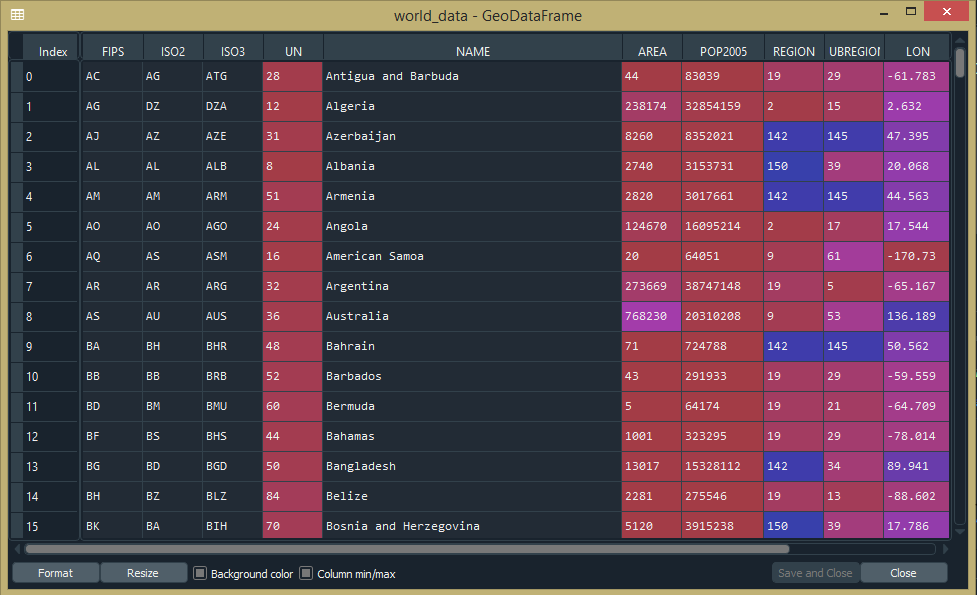## Plotting

If you want to check which type of data you are using then go to the console and type “type(world_data)” which tells you that it’s not pandas data, it’s a geopandas geodata. Next, we are going to plot those GeoDataFrames using plot() method.

Syntax: GeoDataFrame.plot()

Example:

## Python3

 `import` `geopandas as gpd`` ` `# Reading the world shapefile``world_data ``=` `gpd.read_file(r``'world.shp'``)`` ` `world_data.plot()`

Output: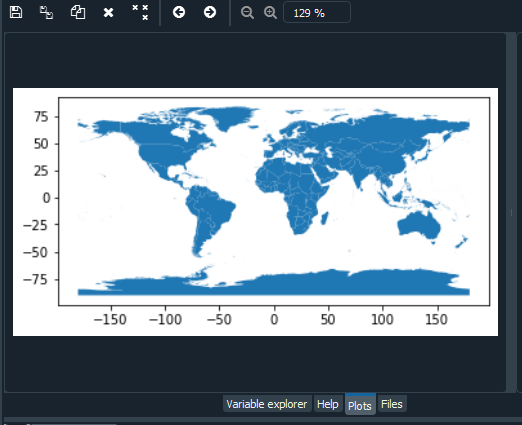## Selecting Columns

If we see the “world_data” GeoDataFrame there are many columns(Geoseries) shown, you can choose specific Geoseries by:

Syntax:

data[[‘attribute 1’, ‘attribute 2’]]

Example:

## Python3

 `import` `geopandas as gpd`` ` `# Reading the world shapefile``world_data ``=` `gpd.read_file(r``'world.shp'``)`` ` `world_data ``=` `world_data[[``'NAME'``, ``'geometry'``]]`

Output: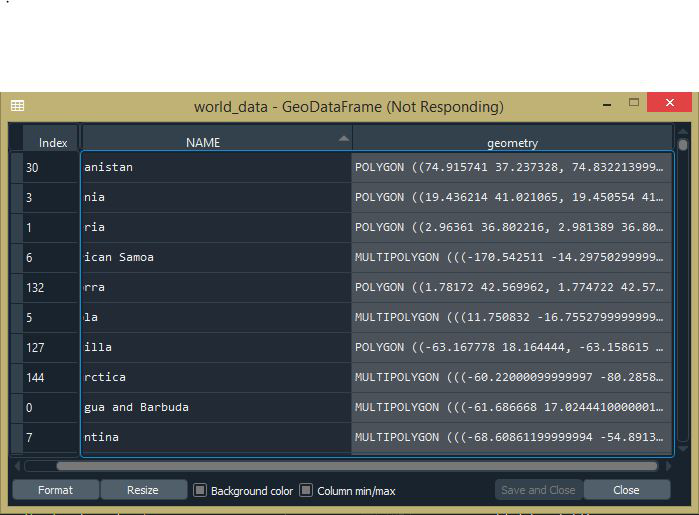## Calculating Area

We can calculate the area of each country using geopandas by creating a new column “area” and using the area property.

Syntax:

`GeoSeries.area`

Returns a Series containing the area of each geometry in the GeoSeries expressed in the units of the CRS.

Example:

## Python3

 `import` `geopandas as gpd`` ` `# Reading the world shapefile``world_data ``=` `gpd.read_file(r``'world.shp'``)`` ` `world_data ``=` `world_data[[``'NAME'``, ``'geometry'``]]`` ` `# Calculating the area of each country``world_data[``'area'``] ``=` `world_data.area`

Output: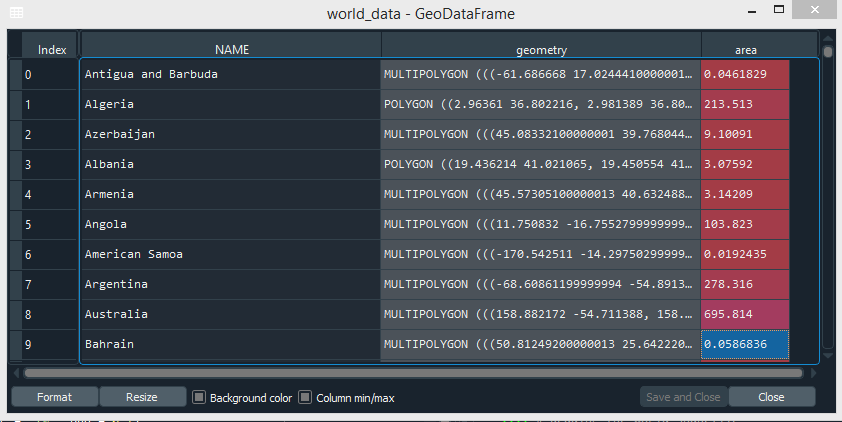## Removing a Continent

We can remove a specific element from the Geoseries. Here we are removing the continent named “Antarctica” from the “Name” Geoseries.

Syntax:

data[data[‘attribute’] != ‘element’]

Example:

## Python3

 `import` `geopandas as gpd`` ` `# Reading the world shapefile``world_data ``=` `gpd.read_file(r``'world.shp'``)`` ` `world_data ``=` `world_data[[``'NAME'``, ``'geometry'``]]`` ` `# Calculating the area of each country``world_data[``'area'``] ``=` `world_data.area`` ` `# Removing Antarctica from GeoPandas GeoDataframe``world_data ``=` `world_data[world_data[``'NAME'``] !``=` `'Antarctica'``]``world_data.plot()`

Output: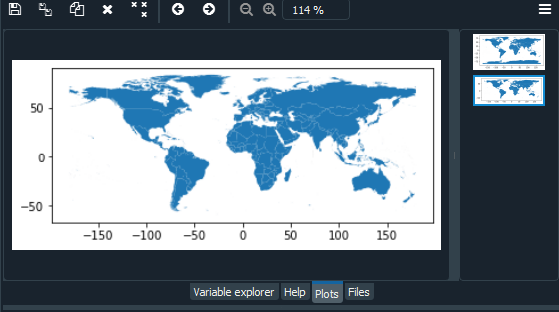## Visualizing a specific Country

We can visualize/plot a specific country by selecting it. In the below example, we are selecting “India” from the “NAME” column.

Syntax:

data[data.attribute==”element”].plot()

Example:

## Python3

 `import` `geopandas as gpd``import` `matplotlib.pyplot as plt ``from` `mpl_toolkits.axes_grid1 ``import` `make_axes_locatable `` ` `# Reading the world shapefile ``world_data ``=` `gpd.read_file(r``'world.shp'``)``world_data ``=` `world_data[[``'NAME'``, ``'geometry'``]]`` ` `# Calculating the area of each country ``world_data[``'area'``] ``=` `world_data.area`` ` `# Removing Antarctica from GeoPandas GeoDataframe``world_data ``=` `world_data[world_data[``'NAME'``] !``=` `'Antarctica'``]``world_data[world_data.NAME``=``=``"India"``].plot()`

Output: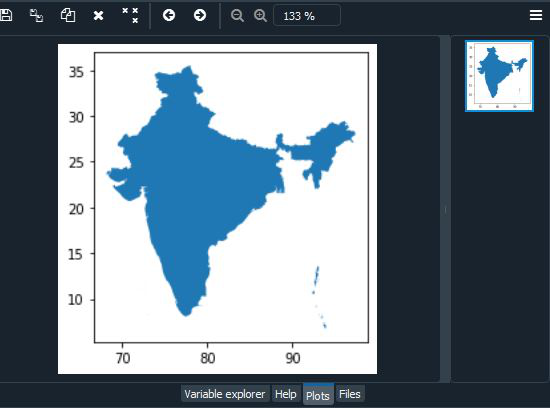## Coordinate Reference System

We can check our current Coordinate System using Geopandas CRS i.e Coordinates Reference System. Also, we can change it to a projection coordination system. The Coordinate Reference System (CRS) is represented as a pyproj.CRS object. We can check current CRS using the following syntax.

Syntax:

GeoDataFrame.crs

to_crs() method transform geometries to a new coordinate reference system. Transform all geometries in an active geometry column to a different coordinate reference system. The CRS attribute on the current GeoSeries must be set. Either CRS or epsg may be specified for output. This method will transform all points in all objects. It has no notion or projecting entire geometries. All segment joining points are assumed to be lined in the current projection, not geodesics. Objects crossing the dateline (or another projection boundary) will have undesirable behavior.

Syntax: GeoDataFrame.to_crs(crs=None, epsg=None, inplace=False)

Parameters

• crs: pyproj.CRS, optional if epsg is specified. The value can be anything accepted by pyproj.CRS.from_user_input(), such as an authority string (eg “EPSG:4326”) or a WKT string.
• epsg: int, optional if crs is specified. EPSG code specifying output projection.
• inplace: bool, optional, default: False. Whether to return a new GeoDataFrame or do the transformation in place.

Example:

## Python3

 `import` `geopandas as gpd`` ` `# Reading the world shapefile``world_data ``=` `gpd.read_file(r``'world.shp'``)`` ` `world_data ``=` `world_data[[``'NAME'``, ``'geometry'``]]`` ` `# Calculating the area of each country``world_data[``'area'``] ``=` `world_data.area`` ` `# Removing Antarctica from GeoPandas GeoDataframe``world_data ``=` `world_data[world_data[``'NAME'``] !``=` `'Antarctica'``]`` ` `# Changing the projection``current_crs ``=` `world_data.crs``world_data.to_crs(epsg``=``3857``, inplace``=``True``)`` ` `world.plot()`

Output: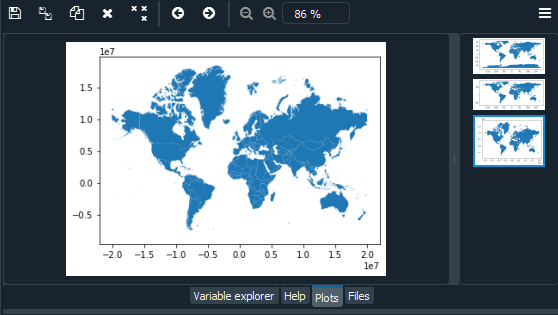## Using Color Maps (cmap)

We can color each country in the world using a head column and cmap. To find out head column type “world_data.head()” in console. We can choose different color maps(cmap) available in matplotlib. In the following code, we have colored countries using plot() arguments column and cmap.

Example:

## Python3

 `import` `geopandas as gpd`` ` `# Reading the world shapefile``world_data ``=` `gpd.read_file(r``'world.shp'``)`` ` `world_data ``=` `world_data[[``'NAME'``, ``'geometry'``]]`` ` `# Calculating the area of each country``world_data[``'area'``] ``=` `world_data.area`` ` `# Removing Antarctica from GeoPandas GeoDataframe``world_data ``=` `world_data[world_data[``'NAME'``] !``=` `'Antarctica'``]`` ` `# Changing the projection``current_crs ``=` `world_data.crs``world_data.to_crs(epsg``=``3857``, inplace``=``True``)`` ` `world_data.plot(column``=``'NAME'``, cmap``=``'hsv'``)`

Output: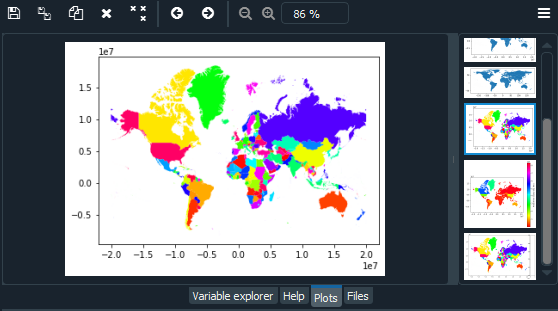Next, we are going to convert the area in sq. km by dividing it to 10^6 i.e (1000000). Output can be seen in variable explorer in the “world_data” variable.

We can add a legend to our world map along with a label using plot() arguments

• legend: bool (default False). Plot a legend. Ignored if no column is given, or if color is given.
• legend_kwds: dict (default None). Keyword arguments to pass to matplotlib.pyplot.legend() or matplotlib.pyplot.colorbar(). Additional accepted keywords when scheme is specified:
• fmt: string. A formatting specification for the bin edges of the classes in the legend. For example, to have no decimals: {“fmt”: “{:.0f}”}.
• labels: list-like. A list of legend labels to override the auto-generated labels. Needs to have the same number of elements as the number of classes (k).
• interval: boolean (default False). An option to control brackets from mapclassify legend. If True, open/closed interval brackets are shown in the legend.

Example:

## Python3

 `import` `geopandas as gpd`` ` `# Reading the world shapefile``world_data ``=` `gpd.read_file(r``'world.shp'``)`` ` `world_data ``=` `world_data[[``'NAME'``, ``'geometry'``]]`` ` `# Calculating the area of each country``world_data[``'area'``] ``=` `world_data.area`` ` `# Removing Antarctica from GeoPandas GeoDataframe``world_data ``=` `world_data[world_data[``'NAME'``] !``=` `'Antarctica'``]``world_data.plot()`` ` `# Changing the projection``current_crs ``=` `world_data.crs``world_data.to_crs(epsg``=``3857``, inplace``=``True``)``world_data.plot(column``=``'NAME'``, cmap``=``'hsv'``)`` ` `# Re-calculate the areas in Sq. Km.``world_data[``'area'``] ``=` `world_data.area``/``1000000`` ` `# Adding a legend``world_data.plot(column``=``'area'``, cmap``=``'hsv'``, legend``=``True``,``                ``legend_kwds``=``{``'label'``: ``"Area of the country (Sq. Km.)"``}, ``                ``figsize``=``(``7``, ``7``))`

Output: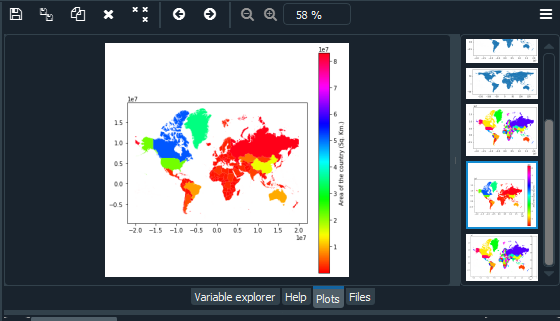## Resizing the legend

We can also resize the legend using ax and cax arguments of plot().

• ax: matplotlib.pyplot. Artist (default None). axes on which to draw the plot.
• cax: matplotlib.pyplot Artist (default None). axes on which to draw the legend in case of color map.

For this, we need matplotlib library.

The axes_divider.make_axes_locatable function takes an existing axes, adds it to a new AxesDivider, and returns the AxesDivider. The append_axes method of the AxesDivider can then be used to create new axes on a given side (“top”, “right”, “bottom”, or “left”) of the original axes. To create axes at the given position with the same height (or width) of the main axes-

Syntax:

position can take any value from: “left”, “right”, “bottom” or “top”.

size and pad should be axes_grid.axes_size compatible.

Example:

## Python3

 `import` `geopandas as gpd``import` `matplotlib.pyplot as plt``from` `mpl_toolkits.axes_grid1 ``import` `make_axes_locatable`` ` `# Reading the world shapefile``world_data ``=` `gpd.read_file(r``'world.shp'``)`` ` `world_data ``=` `world_data[[``'NAME'``, ``'geometry'``]]`` ` `# Calculating the area of each country``world_data[``'area'``] ``=` `world_data.area`` ` `# Removing Antarctica from GeoPandas GeoDataframe``world_data ``=` `world_data[world_data[``'NAME'``] !``=` `'Antarctica'``]``world_data.plot()`` ` `# Changing the projection``current_crs ``=` `world_data.crs``world_data.to_crs(epsg``=``3857``, inplace``=``True``)``world_data.plot(column``=``'NAME'``, cmap``=``'hsv'``)`` ` `# Re-calculate the areas in Sq. Km.``world_data[``'area'``] ``=` `world_data.area``/``1000000`` ` `# Adding a legend``world_data.plot(column``=``'area'``, cmap``=``'hsv'``, legend``=``True``,``                ``legend_kwds``=``{``'label'``: ``"Area of the country (Sq. Km.)"``}, ``                ``figsize``=``(``7``, ``7``))`` ` `# Resizing the legend``fig, ax ``=` `plt.subplots(figsize``=``(``10``, ``10``))``divider ``=` `make_axes_locatable(ax)`` ` `cax ``=` `divider.append_axes(``"right"``, size``=``"7%"``, pad``=``0.1``)``world_data.plot(column``=``'area'``, cmap``=``'hsv'``, legend``=``True``,``                ``legend_kwds``=``{``'label'``: ``"Area of the country (Sq. Km.)"``},``                ``ax``=``ax, cax``=``cax)`

Output: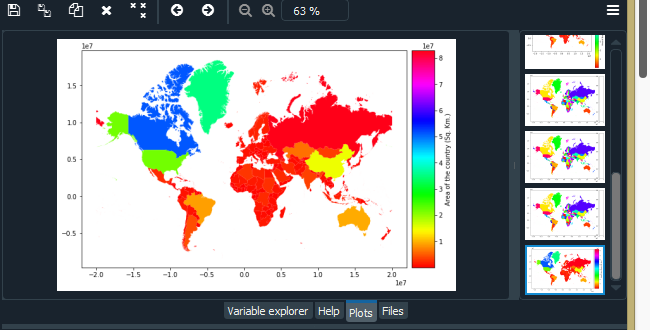## Polyplot and Pointplot Using Geoplot Library

First, we will import Geoplot library. Next, we will load one of the sample datasets(geojson file) present in geoplot. In the below example, we are going to use “world” ,”contiguous_usa”,”usa_cities”,”melbourne” and “melbourne_schools” datasets. List of datasets present in geoplot are mentioned below:

• usa_cities
• contiguous_usa
• nyc_collision_factors
• nyc_boroughs
• ny_census
• obesity_by_state
• la_flights
• nyc_map_pluto_sample
• nyc_collisions_sample
• boston_zip_codes
• boston_airbnb_listings
• napoleon_troop_movements
• nyc_fatal_collisions
• nyc_injurious_collisions
• nyc_police_precincts
• nyc_parking_tickets
• world
• melbourne
• melbourne_schools
• san_francisco
• san_francisco_street_trees_sample
• california_congressional_districts

We can add our own datasets by editing the datasets.py file. Click here for some free sample datasets.

• If you have polygonal data, you can plot that using a geoplot polyplot.
• If your data consists of a bunch of points instead, you can display those points using pointplot.

Syntax :

`geoplot.datasets.get_path(str)`

Syntax for plotting:

```geoplot.polyplot(var)
geoplot.pointplot(var)```

Example:

## Python3

 `import` `geoplot as gplt``import` `geopandas as gpd`` ` `# Reading the world shapefile``path ``=` `gplt.datasets.get_path(``"world"``)``world ``=` `gpd.read_file(path)``gplt.polyplot(world)`` ` `path ``=` `gplt.datasets.get_path(``"contiguous_usa"``)``contiguous_usa ``=` `gpd.read_file(path)``gplt.polyplot(contiguous_usa)`` ` `path ``=` `gplt.datasets.get_path(``"usa_cities"``)``usa_cities ``=` `gpd.read_file(path)``gplt.pointplot(usa_cities)`` ` `path ``=` `gplt.datasets.get_path(``"melbourne"``)``melbourne ``=` `gpd.read_file(path)``gplt.polyplot(melbourne)`` ` `path ``=` `gplt.datasets.get_path(``"melbourne_schools"``)``melbourne_schools ``=` `gpd.read_file(path)``gplt.pointplot(melbourne_schools)`

World Dataset: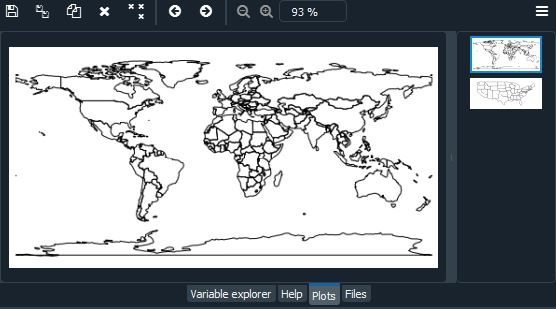USA Dataset: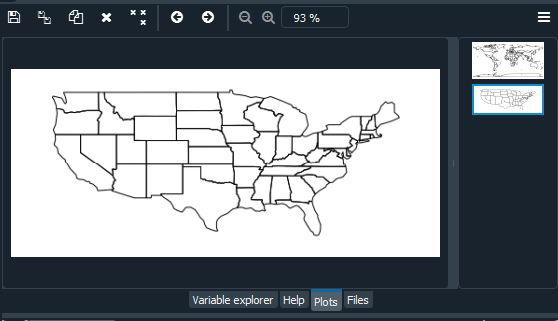USA Cities Dataset: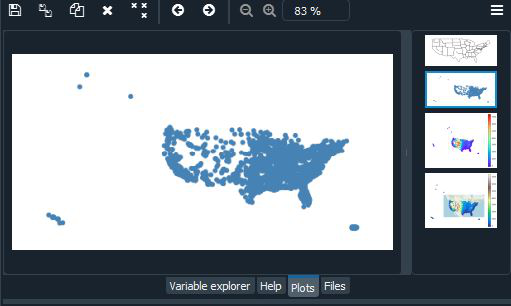Melbourne Dataset: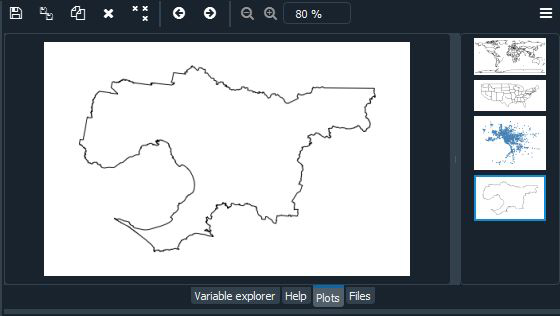Melbourne Schools Dataset: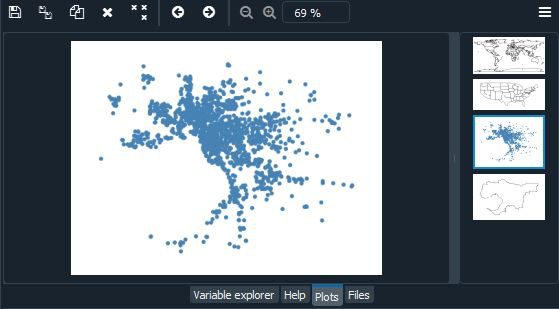We can combine these two plots using overplotting. Overplotting is the act of stacking several different plots on top of one another, useful for providing additional context for our plots:

Example:

## Python3

 `import` `geoplot as gplt``import` `geopandas as gpd`` ` `# Reading the world shapefile ``path ``=` `gplt.datasets.get_path(``"usa_cities"``)``usa_cities ``=` `gpd.read_file(path)`` ` `path ``=` `gplt.datasets.get_path(``"contiguous_usa"``)``contiguous_usa ``=` `gpd.read_file(path)`` ` `path ``=` `gplt.datasets.get_path(``"melbourne"``)``melbourne ``=` `gpd.read_file(path)`` ` `path ``=` `gplt.datasets.get_path(``"melbourne_schools"``)``melbourne_schools ``=` `gpd.read_file(path)`` ` `ax ``=` `gplt.polyplot(contiguous_usa)``gplt.pointplot(usa_cities, ax``=``ax)`` ` `ax ``=` `gplt.polyplot(melbourne)``gplt.pointplot(melbourne_schools, ax``=``ax)`

Output: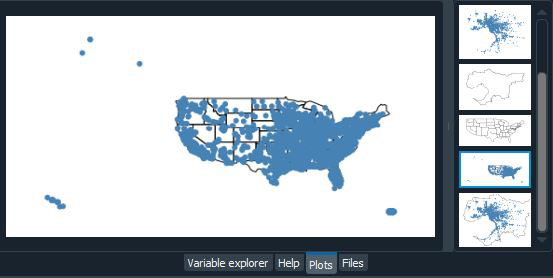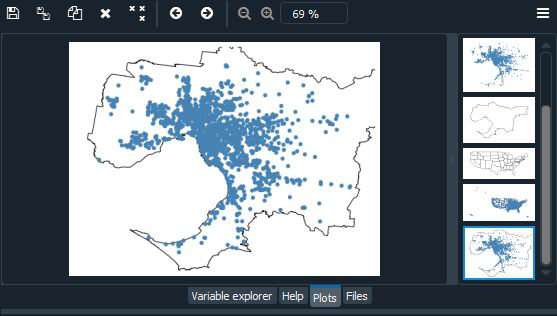You may have noticed that this map of the United States appears to be odd. Because the Earth is a sphere, it is difficult to depict it in two dimensions. As a result, we use some type of projection, or means of flattening the sphere, whenever we take data off the sphere and place it on a map. When you plot data without a projection, or “carte blanche,” your map will be distorted. We can “correct” the distortions by picking up a projection method. Here we are going to use Albers equal-area and WebMercator projection.

Along with this, we are also going to add some other parameters such as hue, legend, cmap, and scheme.

• The hue parameter applies a colormap to a data column.
• The legend parameter toggles a legend.
• Change the colormap using matplotlib’s cmap.
• For a categorical colormap, use a scheme.

Example:

## Python3

 `import` `geoplot as gplt``import` `geopandas as gpd``import` `geoplot.crs as gcrs`` ` `# Reading the world shapefile ``path ``=` `gplt.datasets.get_path(``"contiguous_usa"``)``contiguous_usa ``=` `gpd.read_file(path)`` ` `path ``=` `gplt.datasets.get_path(``"usa_cities"``)``usa_cities ``=` `gpd.read_file(path)`` ` `ax ``=` `gplt.polyplot(contiguous_usa, projection``=``gcrs.AlbersEqualArea())``gplt.pointplot(usa_cities, ax``=``ax, hue``=``"ELEV_IN_FT"``,cmap``=``'rainbow'``, ``               ``legend``=``True``)`` ` `ax ``=` `gplt.webmap(contiguous_usa, projection``=``gcrs.WebMercator())``gplt.pointplot(usa_cities, ax``=``ax, hue``=``'ELEV_IN_FT'``, cmap``=``'terrain'``, ``               ``legend``=``True``)`

Output: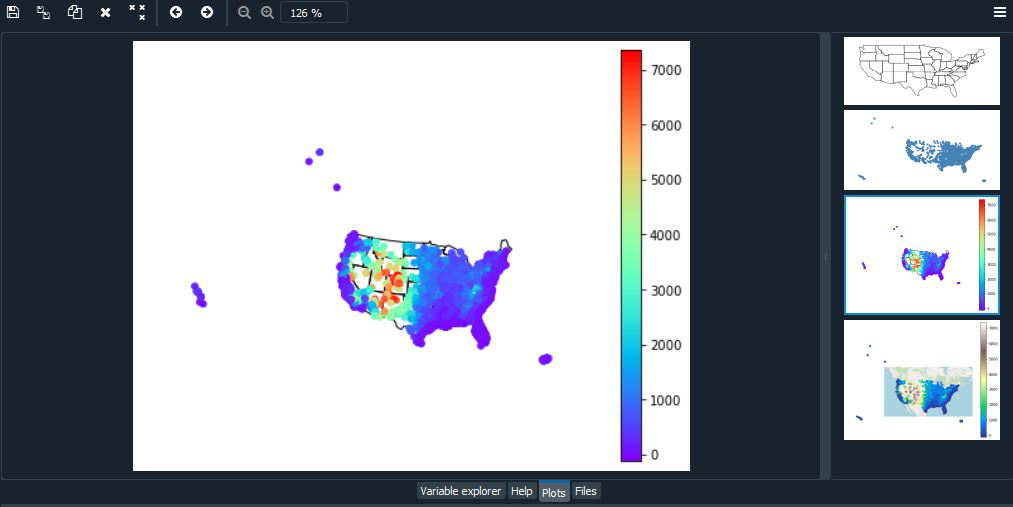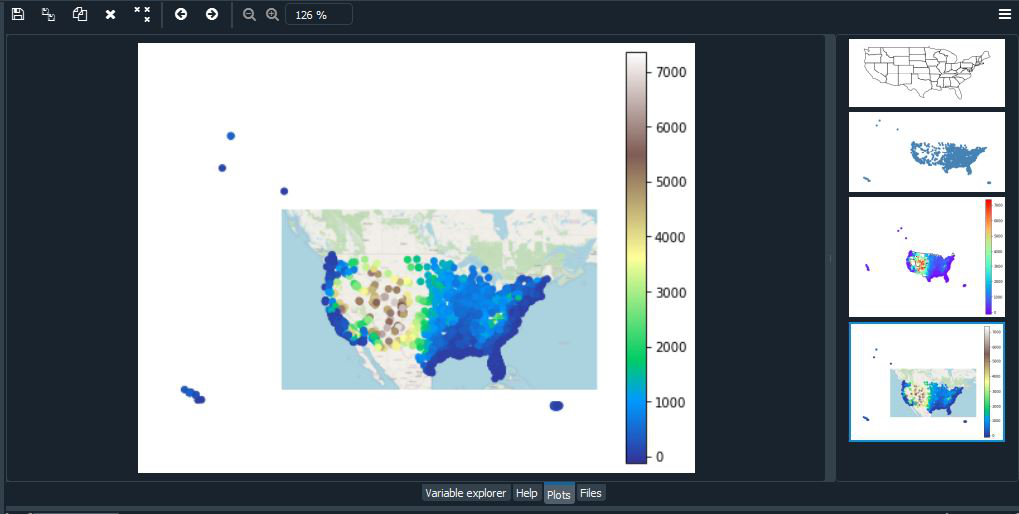## Choropleth in Geoplot

A choropleth takes data that has been aggregated on some meaningful polygonal level (e.g. census tract, state, country, or continent) and uses color to display it to the reader. It’s a well-known plot type, and it’s perhaps the most general-purpose and well-known of the spatial plot types. A basic choropleth requires polygonal geometries and a hue variable. Change the colormap using matplotlib’s cmap. The legend parameter toggles the legend.

Syntax:

`geoplot.choropleth(var)`

Example:

## Python3

 `import` `geoplot as gplt``import` `geopandas as gpd``import` `geoplot.crs as gcrs`` ` `# Reading the world shapefile ``boroughs ``=` `gpd.read_file(gplt.datasets.get_path(``'nyc_boroughs'``))`` ` `gplt.choropleth(boroughs, hue``=``'Shape_Area'``, ``                ``projection``=``gcrs.AlbersEqualArea(), ``                ``cmap``=``'RdPu'``, legend``=``True``)`

Output: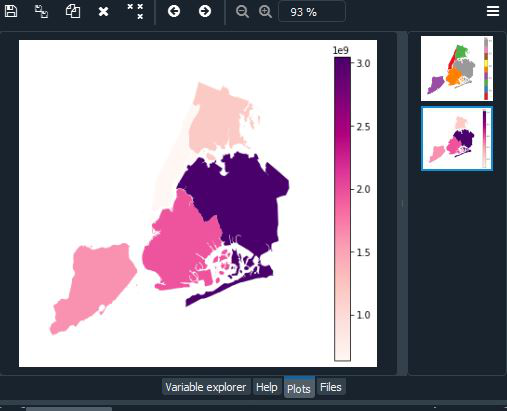To pass the keyword argument to the legend, use the legend_kwargs argument. To specify a categorical colormap, use a scheme. Use legend_labels and legend_values to customize the labels and values that appear in the legend. Here we are going to use mapclassify which is an open-source python library for Choropleth map classification. To install mapclassify use:

• mapclassify is available in on conda via the conda-forge channel:

Syntax:

conda install -c conda-forge mapclassify

• mapclassify is also available on the Python Package Index:

Syntax:

pip install -U mapclassify

Example:

## Python3

 `import` `geoplot as gplt``import` `geopandas as gpd``import` `geoplot.crs as gcrs``import` `mapclassify as mc`` ` `# Reading the world shapefile ``contiguous_usa ``=` `gpd.read_file(gplt.datasets.get_path(``'contiguous_usa'``))`` ` `scheme ``=` `mc.FisherJenks(contiguous_usa[``'population'``], k``=``5``)`` ` `gplt.choropleth(``    ``contiguous_usa, hue``=``'population'``, projection``=``gcrs.AlbersEqualArea(),``    ``edgecolor``=``'white'``, linewidth``=``1``,``    ``cmap``=``'Reds'``, legend``=``True``, legend_kwargs``=``{``'loc'``: ``'lower left'``},``    ``scheme``=``scheme,   legend_labels``=``[``        ``'<3 million'``, ``'3-6.7 million'``, ``'6.7-12.8 million'``,``        ``'12.8-25 million'``, ``'25-37 million'``    ``]``)`

Output: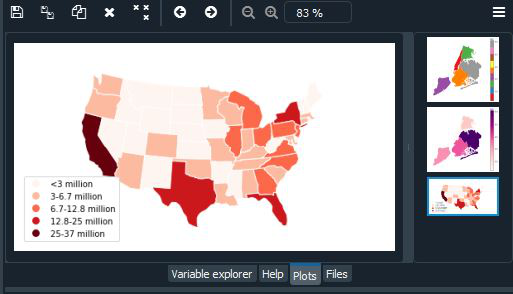## KDE Plot in Geoplot

Kernel density estimation is a technique that non-parametrically estimates a distribution function for a set of point observations without using parameters. KDEs are a popular method for examining data distributions; in this figure, the technique is applied to a geospatial situation. A basic KDEplot takes pointwise data as input.

Syntax:

`geoplot.kdeplot(var)`

Example:

## Python3

 `import` `geoplot as gplt``import` `geopandas as gpd``import` `geoplot.crs as gcrs`` ` `# Reading the world shapefile ``boroughs ``=` `gpd.read_file(gplt.datasets.get_path(``'nyc_boroughs'``))`` ` `collisions ``=` `gpd.read_file(gplt.datasets.get_path(``'nyc_collision_factors'``))`` ` `ax ``=` `gplt.polyplot(boroughs, projection``=``gcrs.AlbersEqualArea())`` ` `gplt.kdeplot(collisions, ax``=``ax)`

Output: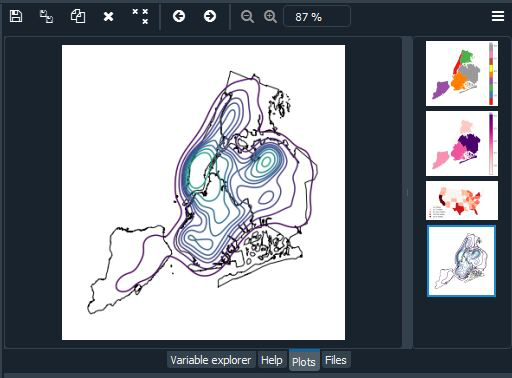## Sankey in Geoplot

A Sankey diagram depicts the flow of information through a network. It’s useful for displaying the magnitudes of data flowing through a system. This figure places the Sankey diagram in a geospatial context, making it helpful for monitoring traffic loads on a road network or travel volumes between airports, for example. A basic Sankey requires a GeoDataFrame of LineString or MultiPoint geometries. hue adds color gradation to the map. Use matplotlib’s cmap to control the colormap. For a categorical colormap, specify the scheme. legend toggles a legend. Here we are using Mollweide projection

Syntax;

`geoplot.sankey(var)`

Example:

## Python3

 `import` `geoplot as gplt``import` `geopandas as gpd``import` `geoplot.crs as gcrs``import` `mapclassify as mc`` ` `# Reading the world shapefile ``la_flights ``=` `gpd.read_file(gplt.datasets.get_path(``'la_flights'``))``world ``=` `gpd.read_file(gplt.datasets.get_path(``'world'``))``scheme ``=` `mc.Quantiles(la_flights[``'Passengers'``], k``=``5``)`` ` `ax ``=` `gplt.sankey(la_flights, projection``=``gcrs.Mollweide(), ``                 ``scale``=``'Passengers'``, hue``=``'Passengers'``, ``                 ``scheme``=``scheme, cmap``=``'Oranges'``, legend``=``True``)`` ` `gplt.polyplot(world, ax``=``ax, facecolor``=``'lightgray'``, edgecolor``=``'white'``)``ax.set_global(); ax.outline_patch.set_visible(``True``)`

Output: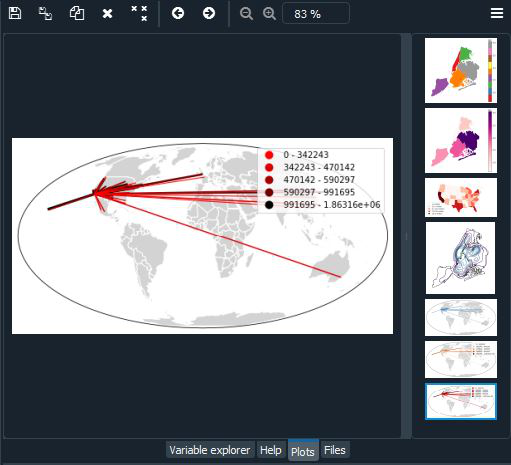My Personal Notes arrow_drop_up